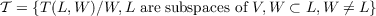#### Vol. 27, No. 2, 1968

 Download this articleFor screen For printingRecent Issues Vol. 325: 1 Vol. 324: 1  2 Vol. 323: 1  2 Vol. 322: 1  2 Vol. 321: 1  2 Vol. 320: 1  2 Vol. 319: 1  2 Vol. 318: 1  2Online Archive Volume: Issue:The Journal Subscriptions Editorial Board Officers Contacts Submission Guidelines Submission Form Policies for Authors ISSN: 1945-5844 (e-only) ISSN: 0030-8730 (print) Special Issues Author Index To Appear Other MSP Journals
Characterizing primes in some noncommutative rings

### Harold G. Rutherford, II

Vol. 27 (1968), No. 2, 387–392
##### Abstract

For a ring R with identity 1, a preprime is a nonempty subset T of R which is closed under the two binary operations, addition and multiplication, of R and with 1T. A prime of R is a preprime of R which is maximal with respect to set inclusion. A field K is locally finite if every member of K is a member of some finite subfield of K. For a finite dimensional vector space V over K let G = HomK(V,V ) denote the full ring of linear transformations of V over K. Let W and L be subspaces of V with W L V aud WL. Let T(L,W) = {α G∕α L W}. Then T(L,W) is a preprime of G. LetWe will show that the primes of G are exactly those preprimes T(L,W) ∈𝒯 with dimKL = 1 + dimKW.

Primary: 16.10
Secondary: 10.00
##### Milestones